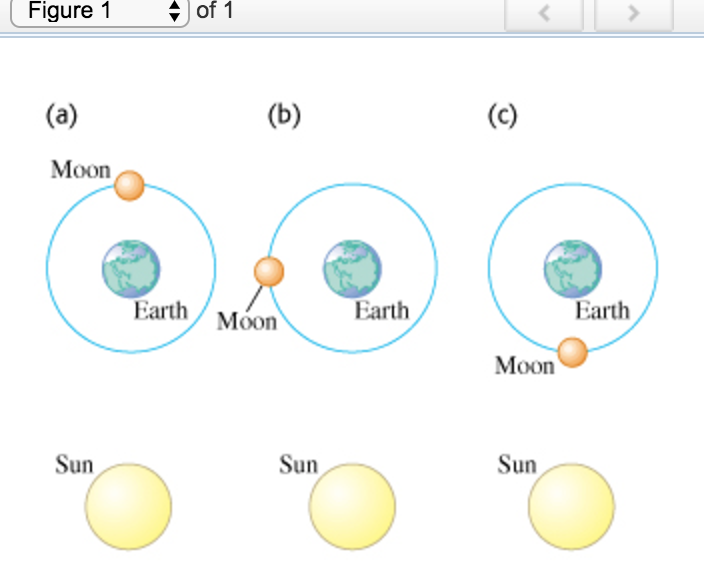# Calculate the magnitude and direction of the net gravitational force on the moon

Calculate the magnitude and direction of the net gravitational force on the moon due to the earth and the sun when the moon is in each of the positions shown in the figure (Figure 1) . (Note that the figure is not drawn to scale. Assume that the sun is in the plane of the earth-moon orbit, even though this is not actually the case.)

Image: